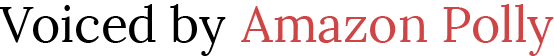# How to Teach Zero and Negative Exponents

 Would You Rather Listen to the Lesson?Sometimes in Math we have things come up that need to be proved but our students do not yet have the skill set mathematically to prove the new concept. This is one of those times. However, we can show them the proof they need for zero and negative exponents using patterns.

## Why is a0 = 1?

Usually when we prove things and  / or use patterns in math class we want to use the simplest example we can. Which in this case would be a base of 2. However, this is one time I do not use 2 as an example. The reason I don't is because powers of 2 and multiplying / dividing by 2 with low numbers are too closely related in their results and can confuse the students (EX: 2x2=4 and 22 =4).

35 = 243
3= 81
327
3= 9
3= 3
3= 1

Notice that when we divide the answers by the base (3) it gives us the following answers.

243/3 = 81
81/3 = 27
27/3 = 9
9/3 = 3
3/3 = 1

If need be you can now use different bases to show the pattern always works.
(Tip: Don't use 10 for the same reason I suggested not to use 2!)

## Patterns with Negative Bases

You can now continue the pattern by showing them negative bases.

(-3)5 = -243
(-3)= 81
(-3)= -27
(-3)= 9
(-3)= -3
(-3)= 1

Notice that when we divide the answers by the base (-3) it gives us the following answers.

(-243)/(-3) = 81
81/(-3) = -27
(-27)/(-3) = 9
9/(-3) = -3
(-3)/(-3) = 1

## Patterns with Negative Exponents

This patter continues on for negative exponents as well.

 33 = 27 32 = 9 31 = 3 30 = 1 3−1= 1/3  3−2 = 1/9 3−3 = 1/27 3−4 = 1/81

So...

1/3 = 1/3
(1/3) / 3 = 1/9
(1/9) / 3 = 1/27
(1/27) / 3 = 1/81

Finally, Make sure to point out that...

1/3 = 1/31
1/9 = 1/32
1/27 = 1/33
1/81 = 1/34
1/243 = 1/35

Now you're ready to move on to Exponents that are Fractions!

Here is your free content for this lesson!

## Zero and Negative Exponents - PDFs

7-1 Guided Notes Teacher Edition - Zero and Negative Exponents (Members Only)

7-1 Lesson Plan - Zero and Negative Exponents (Members Only)

7-1 Online Activities - Zero and Negative Exponents (Members Only)

7-1 Video Lesson - Zero and Negative Exponents (Members Only)

7-1 Project - Zero and Negative Exponents (Members Only)

## Zero and Negative Exponents - Word Docs & PowerPoints

To gain access to our editable content Join the Algebra 1 Teacher Community!
Here you will find hundreds of lessons, a community of teachers for support, and materials that are always up to date with the latest standards.